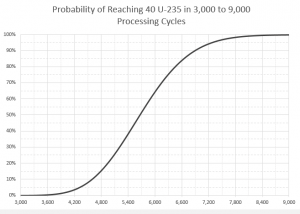This wiki is about 0.17, the current experimental version of Factorio.

Information about 0.16, the current stable version of Factorio, can be found on stable.wiki.factorio.com.

# Uranium processing

Jump to: navigation, search
 Uranium processing Edit
 Prototype type Internal name uranium-processing Required technologies Produced by

Uranium processing is the only way to use uranium ore and the first source of uranium-235 and uranium-238 that is available to the player. The process has a 99.3% chance to produce 1 uranium-238 and a 0.7% chance to produce 1 uranium-235.

## Initiating the kovarex enrichment process

Unlike all other crafting processes in the game, uranium processing creates U-235 and U-238 based on probability, rather than in guaranteed deterministic amounts. Additionally, the Kovarex process requires a bulk stockpile of 40 units of the rare uranium-235 isotope to initiate in a single centrifuge. Therefore, planning uranium mining and processing for the Kovarex process takes certain considerations not encountered elsewhere in the game.

### Expected value

As U-235 is created in centrifuges running uranium processing with p = 0.007, the expected number of processing cycles to gain a single unit is E(1,p) = 1 ÷ p = ~143. The expected number of cycles to gain 40 units is then E(40,p) = 40 × E(1,p) = ~5,714 cycles. Note that running this many cycles requires mining 57,140 uranium ore, a non-trivial task.

However, given the nature of probability, it is impossible to guarantee that 40 units will be reached in this number of cycles; as a matter of fact, some mathematics yields that the probability that 5,714 cycles will produce at least 40 units of U-235 is only about 52%.

### Confidence levelsProbability of reaching 40 U-235 from uranium processing by number of cycles (click to enlarge)

Some further mathematics enables one to calculate the number of uranium processing cycles one must run to obtain at least 40 units of U-235 (given p = 0.007) with a given level of confidence (probability of achieving the set goal).

As discussed in the previous section, the level of confidence corresponding to 5,714 cycles (for which the expected value of U-235 gained is 40) happens to be about 52% (or, in other words, with this number of cycles, one can expect to fail to reach 40 U-235 a bit less than once out of every 2 tries).

Some further example confidence levels that may be of interest to players are given in the table below. Consult the chart to the right for custom values.

Confidence level Failures Cycles required
10% 9 out of 10 4,595
50% 1 out of 2 5,667
90% 1 out of 10 6,894
95% 1 out of 20 7,272
99% 1 out of 100 8,015